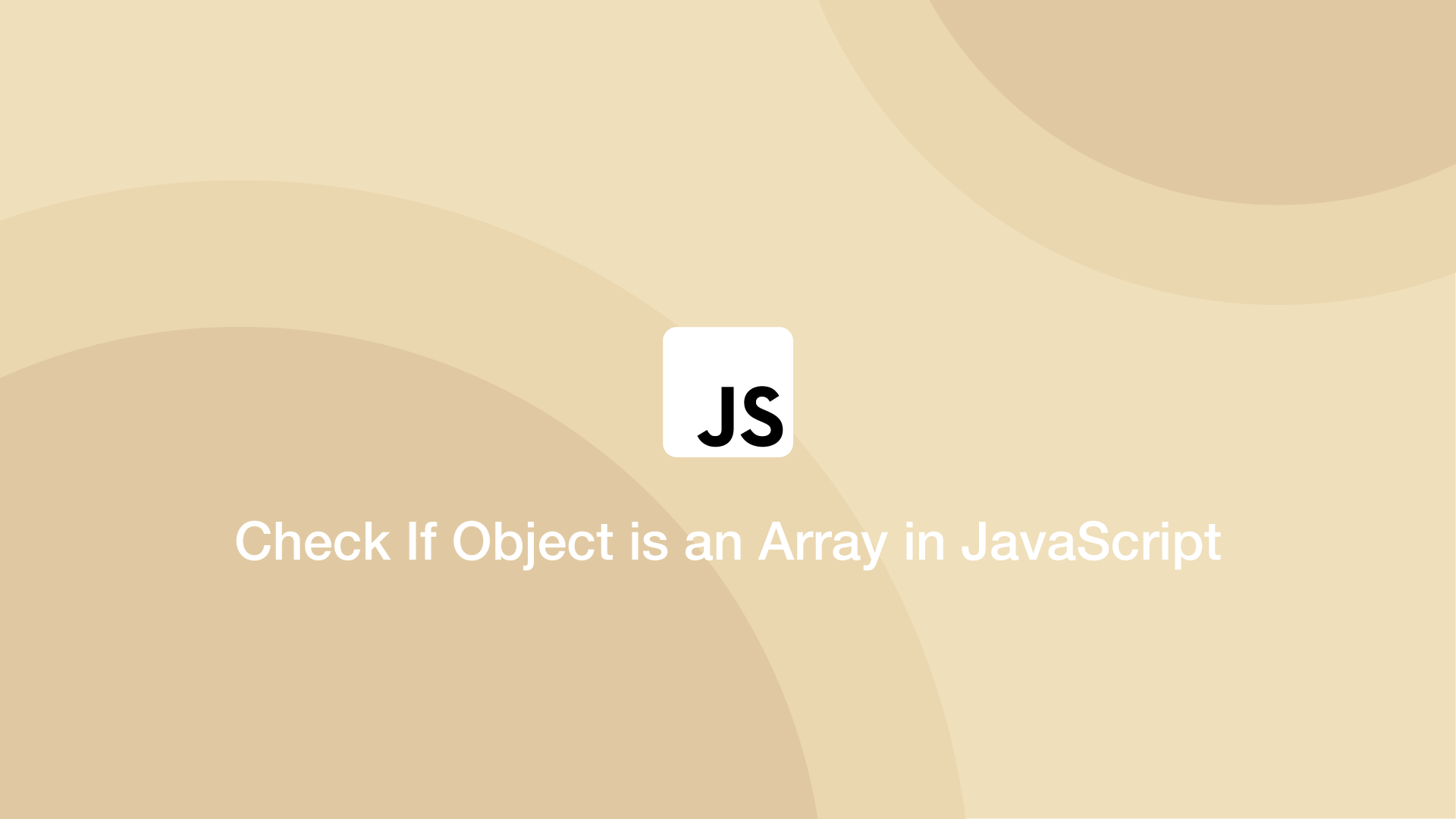# How to Check If Object is an Array in JavaScriptSometimes one needs to check whether a variable is an object or an array in JavaScript. In this tutorial, we will learn how to do that.

## Using the Array.isArray() Method

JavaScript has a built-in method for checking if something is an array called `Array.isArray()`. To use it pass the object to check inside the `()` (parenthesis) of the method.

``````let fruit = ['strawberry', 'orange', 'apple'];

console.log(Array.isArray(fruit));
``````
``````true
``````

`Array.isArray()` returns boolean `true` if the variable is an array and `false` if it is something else.

``````let fruit = null;

console.log(Array.isArray(fruit));
``````
``````false
``````

Here is an example of `if-else` logic which does something if a variable is an array.

``````let fruit = ['strawberry', 'orange', 'apple'];

if (Array.isArray(fruit)) {
// do something with the array.
} else {
// do something if not an array.
}
``````

## Conclusion

You now know how to check if an object is an array in JavaScript.